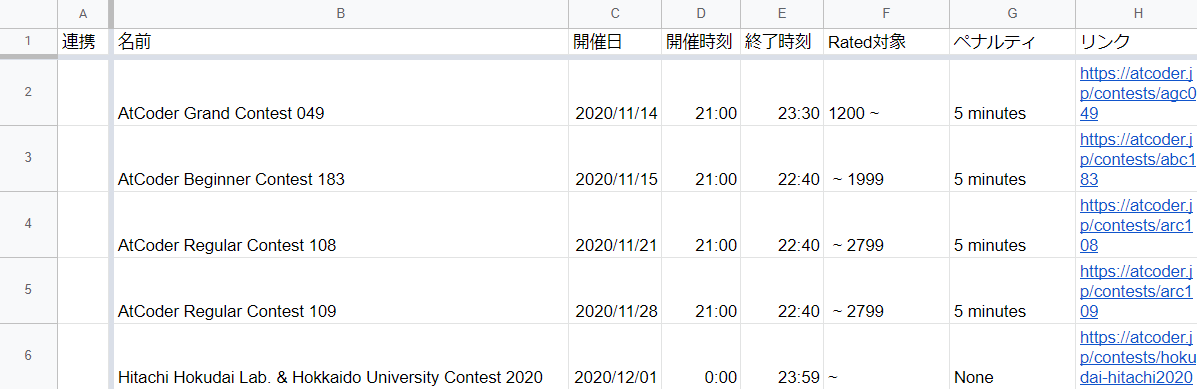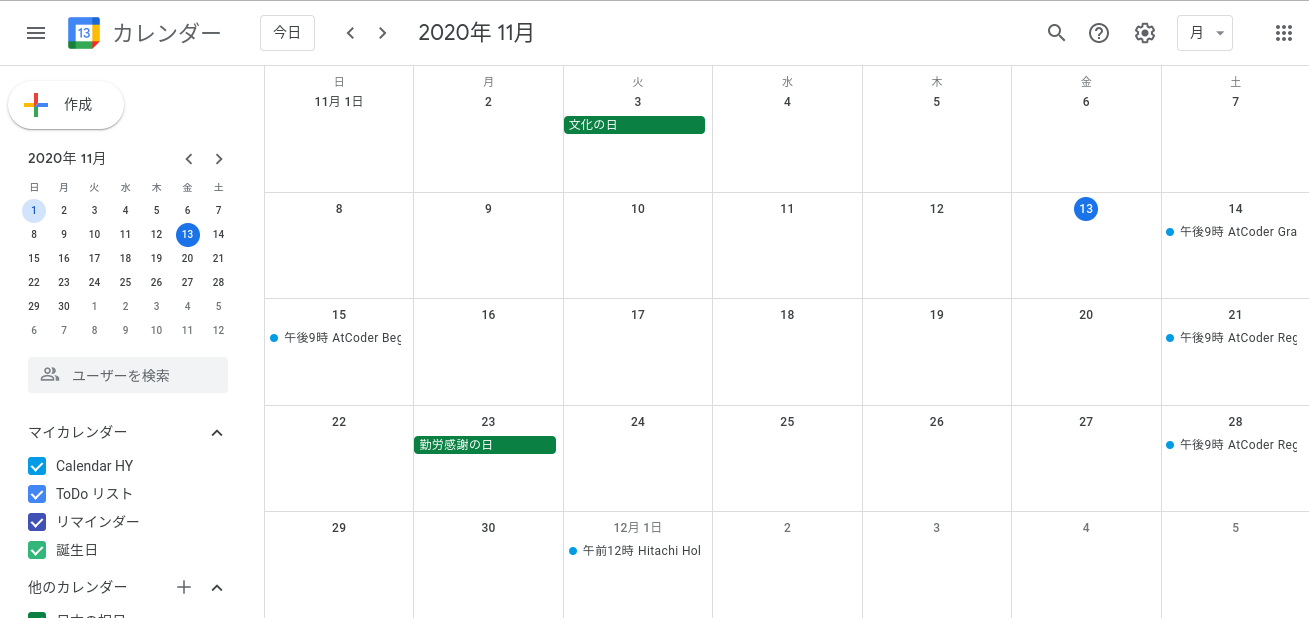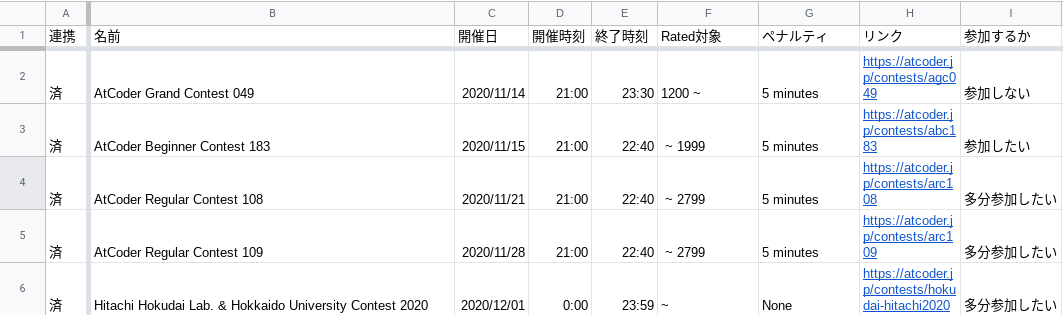# at first

The AtCoder contest is held irregularly, but I sometimes forget to schedule it and it seems to bother my family, so I decided to automate it (I don't know if I can do it). I used Google Colaboratory for execution. Being a beginner in programming, the code can be messy or wrong. sorry.

# From AtCoder to Google Calendar

### procedure

1. Scraping from AtCoder using Python requests, Beautiful Soup, etc.
2. Collect scraped data into Google Spread Sheets using Python's gspread etc.
3. Create an appointment in Google Calendar using GAS from Google SpreadSheets

## 1. Scraping

Actual code Get only the name, date, and link of the AtCoder scheduled contest from the "Contest List" on the AtCoder homepage and make it a list.

``````from bs4 import BeautifulSoup #Import of Beautiful Soup
import requests #Import requests
import datetime
import re

url = "https://atcoder.jp/contests/"
response = requests.get(url).text
soup = BeautifulSoup(response, 'html.parser') #Beautiful Soup initialization
tags = soup.select("tbody a") #Select all a tags under tbody

l=[]
l_n=[]
l_n1=[]

now=datetime.datetime.now().strftime('%Y-%m-%d %H:%M') #Get the current year, month, day, time

for i in tags:
l.append(i.text) #Get the text in the a tag
l.append(i.get("href")) #Get the link attached to the a tag
l.remove("practice contest")
l.remove("/contests/practice")
l.remove("AtCoder Library Practice Contest")
l.remove("/contests/practice2")
l=[l[i:i + 4] for i in range(0,len(l), 4)]
for i in range(len(l)):
l[i]=l[i][0:16]
del l[i]
if (l[i][0:4] > now[0:4]) or (l[i][0:4] == now[0:4] and l[i][5:7] > now[5:7])
or (l[i][0:4] == now[0:4] and l[i][5:7] == now[5:7] and l[i][8:10] >= now[8:10]): #Remove past contests from list
l_n.append(l[i])
for i in range(len(l_n)):
``````

It was difficult to get the start time, end time, rated and penalties of the contest, so I jumped to each link to get it.

``````for i in l_link:
url_n=i
response_n = requests.get(url_n).text
soup_n = BeautifulSoup(response_n, 'html.parser') #Beautiful Soup initialization
tags2=soup_n.select("span.mr-2")+soup_n.select("small.contest-duration") #Get Rated, Penalty, Holding Time, etc.
for j in tags2:
l_n1.append(j.text)
l_n1=[l_n1[i:i + 4] for i in range(0,len(l_n1), 4)]
for i in range(len(l_n1)):
l_n1[i]=l_n1[i][13:]
l_n1[i]=l_n1[i].replace("-","~")
l_n1[i]=l_n1[i][9:]
l_n1[i]=re.sub("\n","",l_n1[i])
l_n1[i]=re.sub("\t","",l_n1[i])
l_n1[i]=l_n1[i][54:60]
del l_n1[i]
for i in range(len(l_n)):
l_n[i]+=l_n1[i]
``````

## 2. Transfer data to spreadsheet

Paste the retrieved data into a spreadsheet.

``````from google.colab import auth
from oauth2client.client import GoogleCredentials

auth.authenticate_user()
worksheet = gc.open('AtCoderNewContestList').get_worksheet(0) #Specify the first sheet of a spreadsheet named AtCoderNewContestList

for i in range(len(l_n)):
if worksheet.update_acell("B"+str(i+2),l_n[i]) in worksheet.range('B2:B10'): #Do not process if the contest has already been added
continue
else:
worksheet.update_acell("B"+str(i+2),l_n[i])
worksheet.update_acell("C"+str(i+2), l_n[i][0:4]+"/"+l_n[i][5:7]+"/"+l_n[i][8:10])
worksheet.update_acell("D"+str(i+2),l_n[i][11:])
worksheet.update_acell("E"+str(i+2),l_n[i][-1])
worksheet.update_acell("F"+str(i+2),l_n[i])
worksheet.update_acell("G"+str(i+2),l_n[i])
worksheet.update_acell("H"+str(i+2),"https://atcoder.jp"+l_n[i])
worksheet.update_acell("A"+str(i+2),"")
``````

The result is below.It's working pretty well.

## 3. Add appointment with GAS

Add appointments to Google Calendar using GAS. Due to various reasons, I decided to create a new Google account, access the created spreadsheet, extract data with GAS, and create a schedule. I can no longer access the account I created at the beginning. why? You can write GAS code by selecting "Script Editor" from "Tools" in the spreadsheet. (GAS can understand the contents of the code but cannot write it, so I copied and edited the code on the site below.) (Reference: https://qiita.com/cazimayaa/items/5fdfbc060dff7a11ee15)

``````
function myFunction() {
//Get the sheet of the currently selected spreadsheet
var sheet = SpreadsheetApp.getActiveSheet();
//Get the contents of the cell from the obtained sheet
var values = sheet.getDataRange().getValues();
var calendar = CalendarApp.getDefaultCalendar();
//* The 0th part of var i is the header, so start from 1.
for (var i = 1; i < values.length; i++) {
var status = values[i];
if (
) {
//scheduled date
var date = values[i];

//Start time
var startTime = values[i];
var startDateTime = new Date(date.getFullYear(),
date.getMonth(),
date.getDate(),
startTime.getHours(),
startTime.getMinutes(), 0);
//ending time
var endTime = values[i];
var endDateTime = new Date(date.getFullYear(),
date.getMonth(),
date.getDate(),
endTime.getHours(),
endTime.getMinutes(), 0);
//title
var title = values[i]+values[i];
var options = {
description: values[i]
}
// var event = calendar.createEvent(title, startDateTime, endDateTime);
//Just add options to the argument
var event = calendar.createEvent(title, startDateTime, endDateTime, options);
//Register to calendar
After execution calendarSpreadsheetI did it.